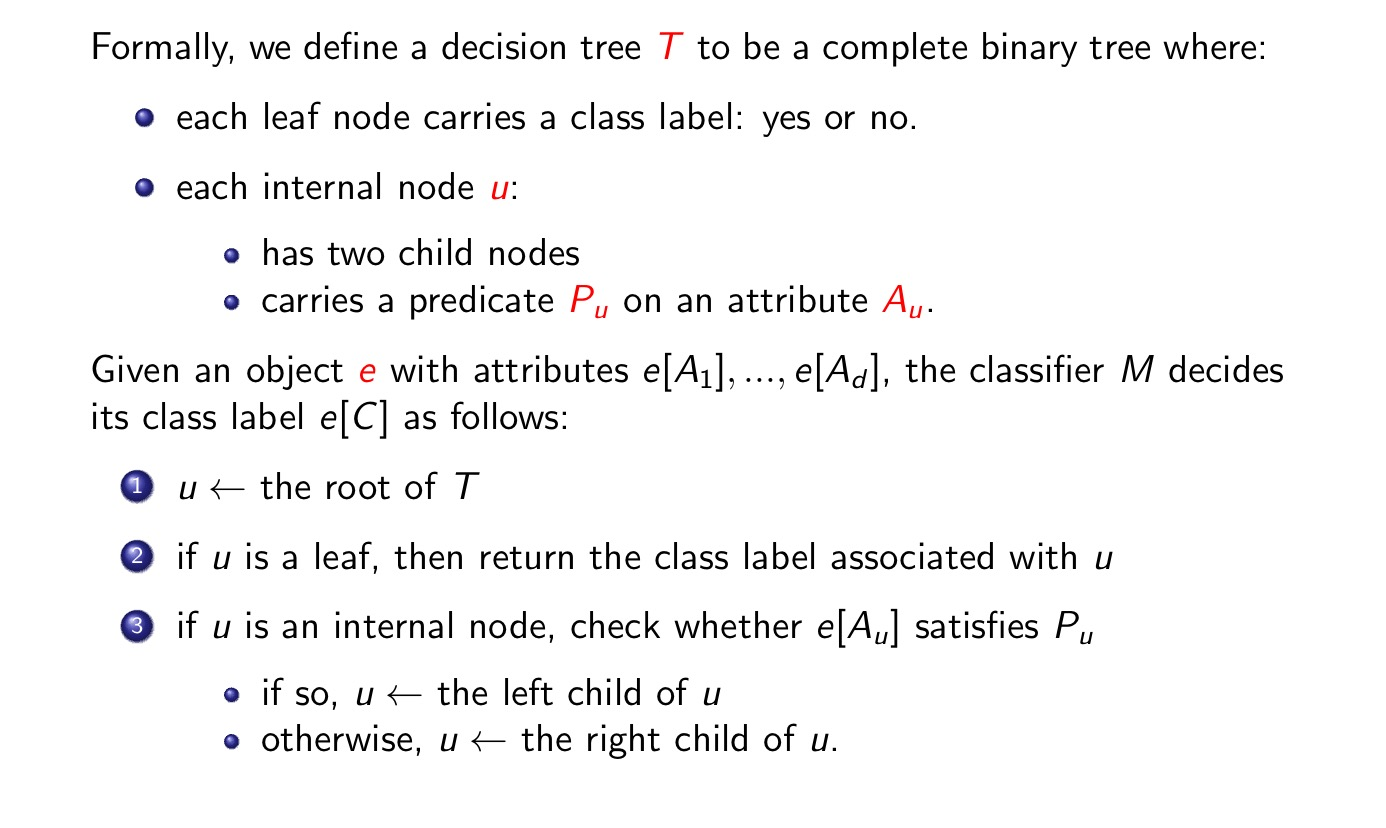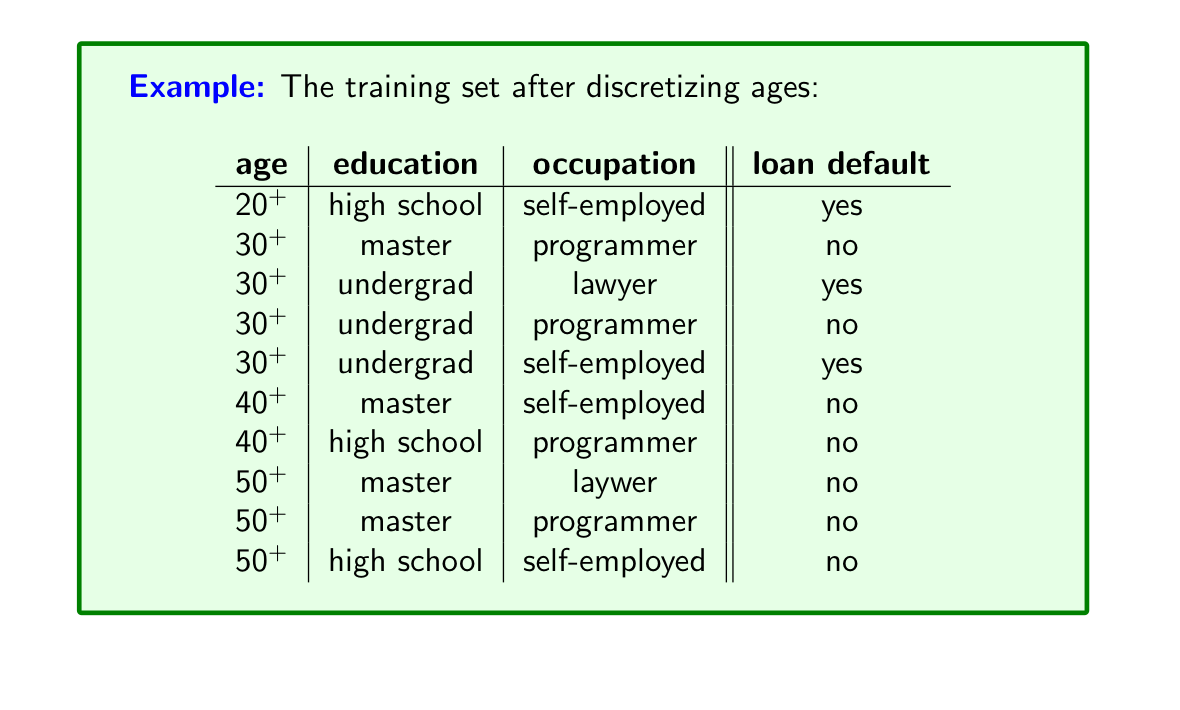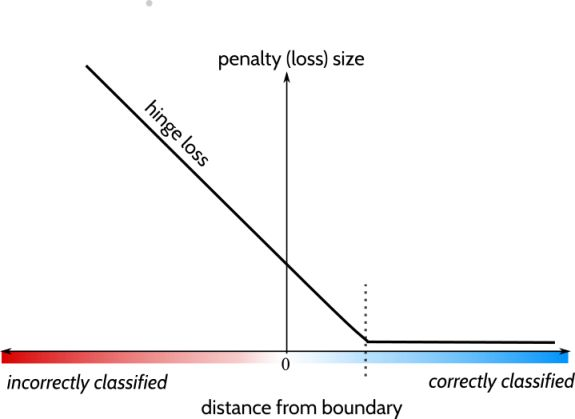## 决策树## 贝叶斯分类## SVM(支持向量机)

$D_0$和$D_1$ 是 n 维欧氏空间中的两个点集。如果存在 n 维向量 w 和实数 b，使得所有属于$D_0$的点$x_i$都有$wx_i + b > 0$， 而对于所有属于$D_1$的点$x_j$ 则有$wx_j + b < 0$，则我们称 $D_0$ 和 $D_1$线性可分。将$D_0$和$D_1$完全正确地划分开的$wx + b = 0$ 就成了一个超平面。

SVM的目的

## Perceptron(感知机)

### 感知机的学习算法

#### 原始形式：

$\eta (0 < \eta \leq 1)$是步长，也就是学习率。

1.$\mathbf{w} \leftarrow 0$, $b \leftarrow 0$
2.在训练集中选取数据$(x_i,y_i)$
3.如果 $y_i(\mathbf{w} \cdot x_j + b) \leq 0$

4.转到2不断重复，直到训练集里面没有误分类的点。

#### 感知机算法的对偶形式

1.$\mathbf{w} \leftarrow 0$, $b \leftarrow 0$
2.在训练集中选取数据$(x_i,y_i)$
3.如果 $y_i(\sum\limits_{j=1}^{N} w_j y_j x_j \cdot x_{i} + b) \leq 0$

4.转到2不断重复，直到训练集里面没有误分类的点。

sign函数，符号函数，对于$x \geq 0$, $sign(x)=1$; 对于对于$x < 0$, $sign(x)=-1$;

## SVM与Perceptron的区别

1.普通的感知机不能产生大间隔(margin),但是SVM可以。
2.加入了L2正则化的带margin的感知机基本就是SVM。

Perceptron对应的优化问题：

SVM（soft margin）对应的优化问题：

SVM和perceptron区别之一是SVM使用了hinge损失函数，而perceptron采用0/1损失函数，分对了就没损失。这个改进使得感知器具备了产生大间隔的潜质，hinge loss不仅要分类正确，而且置信度足够高的时候，损失才为0，对学习有更高的要求。其实这个道理和SVM中的margin是相符合的，不仅要分类正确，还要使得margin最大化。后面部分加上的是L2正则化，避免产生无意义的大函数间隔，而是产生大的几何间隔。

Hinge Loss 在二分类情况下，公式如下：Hinge loss的分类器预测值 $\hat{y} \in R$。$|\hat{y}|$越大，说明样本点离分割超平面越远，即该样本点很容易被分类。所以选择合适的损失函数进行优化的时候，其实没必要关注离超平面很远的样本。为此，我们可以通过对距分离超平面的距离选择一个阀值，来过滤掉这些离超平面很远的样本。这就是Hinge loss的另一个精髓。$L(y) = max(0, 1-y \cdot \hat{y})$。这里面的1就是选择的的阀值。

## 大数据知识点

minHash(最小哈希)和LSH(局部敏感哈希)

https://blog.csdn.net/liujan511536/article/details/47729721

https://www.jianshu.com/p/a83a52da14a2?open_source=weibo_search

https://blog.csdn.net/zhoujing_0424/article/details/50817468

apriori 算法

https://www.edureka.co/blog/apriori-algorithm/

https://www.jianshu.com/p/2ee0a247a8cc

bloomfilter

https://llimllib.github.io/bloomfilter-tutorial/zh_CN/

https://blog.csdn.net/llwszjj/article/details/25629009

https://my.oschina.net/hunglish/blog/787596

https://blog.csdn.net/sherrylml/article/details/49363509

https://blog.csdn.net/bitcarmanlee/article/details/52662518

SVD - 奇异值分解

https://zhuanlan.zhihu.com/p/26614750

PMF

https://blog.csdn.net/shenxiaolu1984/article/details/50372909

pagerank